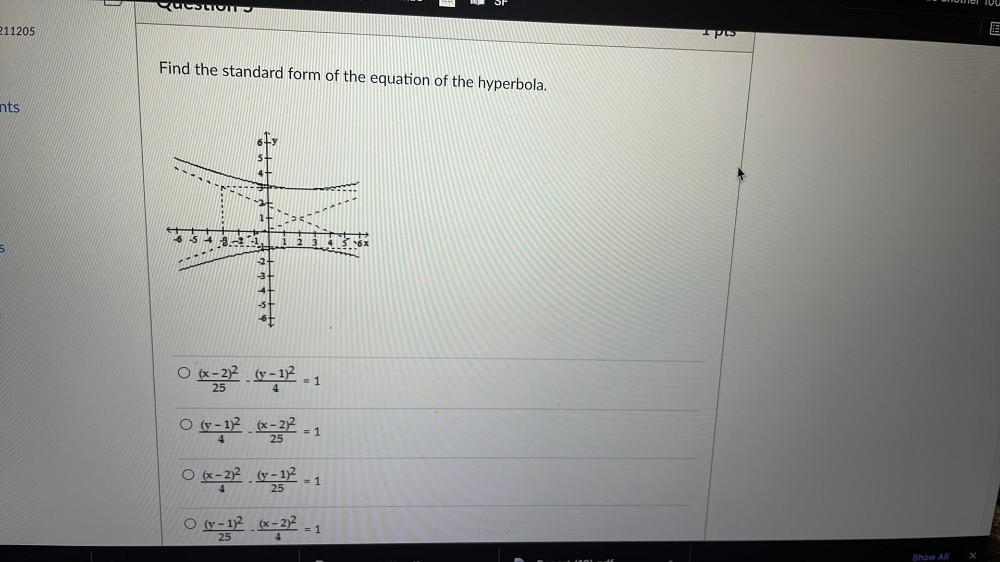Question:

# SE Unuel JUU B QUESTION 211205 Ipes Find the standard form of the equation of the hyperbola. nts 6 5+ 6 5 4 3.-25 1 2 3 4 5 6 důSE Unuel JUU B QUESTION 211205 Ipes Find the standard form of the equation of the hyperbola. nts 6 5+ 6 5 4 3.-25 1 2 3 4 5 6 důlu. = 1 4 = 1 4 25 O (x - 2)2 (v - 1)2 =332 0-12 . 25 O (y - 1)2 (x - 2)2 132.67272 - 1 O (x - 2)2 (y - 12 = 1 O (y - 1)2 (x - 2)2 (? 6-232 - 1 25 = 1 4 25 = 4 Show All X